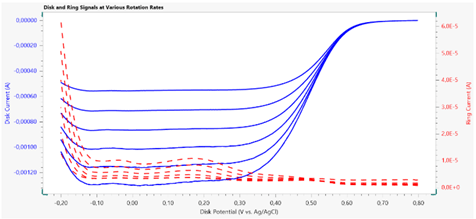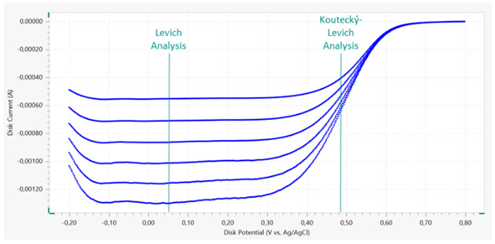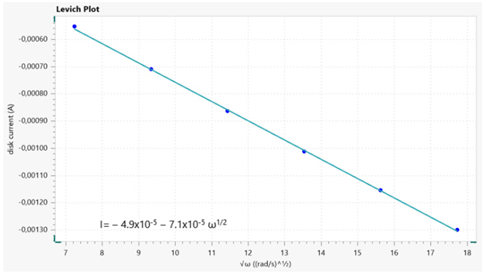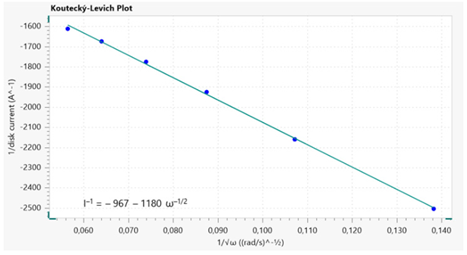# Using a Rotating Ring Disk Electrode to perform the Oxygen Reduction Reaction

The field of electrocatalysis is dependent upon the oxygen reduction reaction (ORR) in many ways, which explains the enormous amount of research that has been conducted on it. In an aqueous acidic electrolyte, the pathway adopted by the ORR depends on the conditions under which the reaction proceeds.

The most commonly targeted outcome is the 4-electron process in equation 1, but the other equations show various other routes, including the formation of H2O2 as an intermediate compound or incomplete reduction.

 O2 + 4H+ + 4e-→ 2H2O (1)
 O2 + 2H+ + 2e- → H2O2 (2)
 H2O2 + 2H+ + 2e- → 2H2O (3)
 2H2O2 → 2H2 + O2 (4)

Hydrogen peroxide formed during the ORR at any stage may be electrochemically identified by its oxidation reaction as in equation 5:

 H2O2 → 2H2O + O2 (5)

In most cases the ORR is performed under hydrodynamic conditions, where the working electrode (WE) is rotated to ensure that forced convection occurs inside the electrochemical cell.

The advantage is a stable current density limited by mass transfer because of the movement, which might not otherwise occur in a sustained fashion because of the slow reaction characteristics. The current experiment used a rotating ring disc electrode (RDDE) for the ORR.

## Experimental Conditions

For this experiment, an Autolab PGSTAT302N potentiostat/galvanostat equipped with a dual-mode bipotentiostat (the BA module), was used with the Autolab RRDE hardware.

The Autolab RRDE cell has a four-electrode setup. Only very clean cell components were allowed to be in contact with the RDDE to avoid vitiating the electrochemical reactions at the Pt electrode, which is notoriously easy to deflect by impurities present within the system.

The cleaning was done by soaking each component in diluted sulfuric acid and hydrogen peroxide solution. They were then rinsed repeatedly in boiling water. Two working electrodes, the Pt disk (WE1) and the Pt ring (WE2) were used in one RRDE tip, which is reported to have a theoretical collection efficiency of 24.9%, calculated on the basis of ring and disk geometry.

The experimental collection efficiency value and the practical importance of the theoretical collection efficiency cannot be explained in detail here. The counter electrode (CE) was a platinum sheet with a double junction Ag/AgCl electrode being the reference electrode (RE). The inner compartment of the cell contained 3M aqueous potassium chloride while the outer compartment contained 0.5M sulfuric acid (the working electrolyte).

The CE and RE were both placed a little above the lowest part of the RDDE tip which was inside the cell. This was to keep the hydrodynamics unchanged. About 15 minutes prior to the start of the experiment, the working electrolyte was purged using high purity oxygen gas so that it was saturated with dissolved oxygen. Again, during the measurement, an oxygen gas blanket was maintained to cover the surface so that the dissolved oxygen concentration remained constant.

The procedure used is called “hydrodynamic linear sweep with RDDE” and the Nova 2.1 software was utilized to apply it. At different rates of electrode rotation (ω) linear voltammetry staircase sweeps were conducted at the disk, from 0.70 to -0.20 V, at a scan rate of 50 mV/s, but at the ring, a fixed 1.00 V potential was maintained, and the current flow in response to the sweeps was recorded at the same rotation values.

## Experimental Results

The plots of a typical ORR RDDE experiment (Figure 1) show that the value of ω went from 500 to 3000 RPM, being selected with an eye to being easily represented on the Levich plot, and therefore having a square root relationship. As the potential at the disk goes from high to low, the ORR begins and is reflected as a negative signal, observed from about 0.65 mV.Figure 1. Plot of the disk (solid lines) and ring (dashed lines) data collected during the hydrodynamic RRDE study of the ORR using a Pt disk and Pt ring. The Pt disk potential was swept at a scan rate of 50 mV s–1; the ring potential was fixed at 1.00 V vs. Ag/AgCl. Electrode rotation rates between 500 and 3000 RPM were applied.

The current increases in proportion to the drop in potential and flattens out at E=-0.20 to -0.10 V. This plateau phase is the mass transfer limited region, where the voltammetry plot shows the rate of ORR to proceed in direct relation to oxygen availability at the surface of the electrode.

As the ω goes up so does the strength of the cathodic current, reflecting the Levich plot. When the potential drops lowest (below -0.10 V), the cathode current also drops because of hydrogen adsorption on the surface of the Pt electrode, disrupting the ORR. Since the ring always has a fixed potential of 1.00 V, it is not plotted.

The current does change at the ring, however, with the sweeps, because of the closeness of the two electrodes at only 375 µm distance and therefore sharing of the chemical changes at the disk by the ring. As the reaction proceeds, reaction products such as H2O2 will be found in proximity to the ring as well by simple diffusion, and may react still more, giving rise by oxidation to an anodic current at the ring.

Thus, such a finding indicates the production of H2O2 at the disk. As the disk potential sweep reaches low values the ORR becomes significant and anodic current increases at the ring. At the plateau phase, with disk current being limited by mass transfer, this current is stable, but increases steeply once the cathodic current decreases because of hydrogen being adsorbed on the disk surface. This is because the ORR has shifted to favoring H2O2 production.Figure 2. Linear sweep voltammetry profiles (top) from the disk electrode at various rotation rates; the potential value for the Levich and Koutecký-Levich analyses are indicated.  Levich (middle) and Koutecký-Levich (bottom) plots.

The Levich and Koutecký-Levich plots (Figure 2) for the ORR will reflect the change in current with electrode rotation rate when the potential is kept constant. If the potential is chosen from the plateau region of the I vs E curves, the Levich plot will become linear according to the equation below:

 il = 0.62 x AnFD2⁄3ν-1⁄6C√ω (6)

If the potential is from the area in which the current flow is influenced by kinetic and mass transfer, the Koutecký-Levich plot is linear following the next equation:

 O2 + 4H+ + 4e-→ 2H2O (7)

For both equations above, the variables are:

A is the geometric disk area in (cm2)

F is Faraday’s constant (C mol-1)

D is the diffusion coefficient of O2 in the electrolyte (cm2 s-1)

ν is the kinematic viscosity of the electrolyte (cm2 s-1)

C is the concentration of O2 in the electrolyte (mol cm-3)

ω is the angular frequency of rotation (rad s-1)

n is the number of electrons involved in the reaction

Both the Levich and Koutecký-Levich plots can be used to find slopes and intercepts by the use of linear regression. The latter plot yields the kinetic current ik, from the y-intercept which is the reciprocal of this value. The ik value can be applied in equation 8 to find the rate constant for electron transfer, k, provided the number of electrons taking part in the reaction is known.

 ik=nFAkC (8)

At any potential the primary mechanism of reaction may be found by detecting hydrogen peroxide at the ring electrode (WE2). In the mass transfer plateau phase, ORR uses both the four-electron and two-electron pathways but when the potential drops below -0.10 V the increase in H2O2 at the ring shows the two-electron pathway is dominating the reaction.

The Levich plot can also be subjected to linear regression to get the slope value, which will help calculate the diffusion coefficient of oxygen in the system. Table 1 shows the variables in this system, assuming the oxygen concentration in the electrolyte to be at saturation level.

In the current experiment the diffusion coefficient for oxygen in electrolyte is 7.6 x 10–5 cm2 s–1 with the four-electron mechanism, and 2.2 x 10–5 cm2 s–1 when the two-electron pathway is dominant. The measured signal from the electrochemical signal reflects both these processes.

This value is higher than that seen in literature (about 1.4 x 10–5 cm2 s–1). This may possibly be explained by the extreme sensitivity of this parameter to the oxygen concentration in the electrolyte, which introduces uncertainty into the measurement.

Table 1. System variables for the oxygen reduction reaction in 0.5 M H2SO4 electrolyte at room temperature.

Parameter Value Unit
Kinematic viscosity 0.010 cm2 s–1
Solubility of O2 1.1 x 10–6 mol cm–3
Electrode geometric area 0.20 cm2

## Conclusions

In this article, the Autolab RRDE system is utilized to conduct the ORR using a Pt disk/ring electrode. The hydrogen peroxide at the disk during the reaction is found at the ring, and serves to indicate the dominant path of reaction.

The Levich and Koutecký-Levich plots are fitted to the data with the help of linear regression. This gives various equations which are useful in finding the diffusion coefficients of oxygen in the electrolyte, the number of electrons transferred during the ORR and the rate constant for electron transfer.

## References

1. N.A. Anastasijević et al. J. Electroanal. Chem. 229 (1987) 305
2. Nenad M. Marković et al. J. Phys Chem. 99 (1995) 3411
3. Carlos M. Sánchez- Sánchez and Allen J. Bard. Anal. Chem. 81 (2009) 8094
4. Francisco J. Vidal-Iglesias et al. Electrochem. Commun. 15 (2012) 42.
5. Alfred B. Anderson. Electrocatal. 3 (2012) 176.
6. K-L. Hsueh et al. Electrochimica Acta. 28 (1983) 691.This information has been sourced, reviewed and adapted from materials provided by Metrohm AG.

## Citations

• APA

Metrohm AG. (2020, May 18). Using a Rotating Ring Disk Electrode to perform the Oxygen Reduction Reaction. AZoM. Retrieved on October 22, 2020 from https://www.azom.com/article.aspx?ArticleID=15450.

• MLA

Metrohm AG. "Using a Rotating Ring Disk Electrode to perform the Oxygen Reduction Reaction". AZoM. 22 October 2020. <https://www.azom.com/article.aspx?ArticleID=15450>.

• Chicago

Metrohm AG. "Using a Rotating Ring Disk Electrode to perform the Oxygen Reduction Reaction". AZoM. https://www.azom.com/article.aspx?ArticleID=15450. (accessed October 22, 2020).

• Harvard

Metrohm AG. 2020. Using a Rotating Ring Disk Electrode to perform the Oxygen Reduction Reaction. AZoM, viewed 22 October 2020, https://www.azom.com/article.aspx?ArticleID=15450.

## Tell Us What You Think